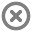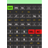Loading...

### Organization and Device Administration## Info## Error# Kal Scientific Calculator

by LearnPad Education Mexico

Application (628kB)

Free

## Description

Scientific calculator with all the functions, easy to use, includes a display for writing operations without character limit allows use parentheses and respects the hierarchy of the operations and functions, the result is displayed on the second line of the display with the ability to modify or correct an action.

Scientific Calculator Features
* Allows function graphs
* Feature (FML), 100 built-in formulas
* Typical operations (add, subtract, multiply, divide).
* The root and exponential functions (nth, nth root, square, square root, cube root)
* Logarithmic Functions (log10, ln, powers of 10, exp)
* Trigonometric functions (sin, cos, tan, including inverse and hyperbolic)
* Three angle modes (DEG, RAD, GRA)
* Random number generator
* PI
* Permutation (nPr) and combinations (nCr)
* Absolute value factor.
* Allows numbers in scientific notation.
* Includes major scientific constants.
* Basic and converting number systems (decimal, hexadecimal, octal, binary) Operations
* Set number of decimal and thousands separator.
* History of the last 10 operations and results.
* Allows to store up to 10 different results

Statistical Mode

* Standard deviation
* Arithmetic
* Sum of values